# What is Euler’s Formula?

First, let’s write Euler’s formula itself.

$e^{i\theta} = \cos(\theta) + i\sin(\theta)$

As you can see in equation (1), Euler’s formula consists of several elements, and the most unique one among them is the imaginary exponent.

In this article, let’s explore the meaning of this seemingly complex equation.

# Geometric Meaning

If we take a closer look at Euler’s formula, we can see that it contains the natural constant $e$, the imaginary number $i$, and the trigonometric functions $\cos$ and $\sin$.

At first glance, it may be difficult to extract any information from the left-hand side with the imaginary exponent. Therefore, let’s try to understand the formula’s meaning from the right-hand side.

## From the right-hand side of the formula

Rewriting the right-hand side of equation (1), we have:

$\cos(\theta) + i \sin(\theta)$

If we let $\cos(\theta)$ and $\sin(\theta)$ be represented by $x$ and $y$, respectively, then equation (2) is simply equal to $x + iy$.

In other words, if we think about the meaning of Euler’s formula from the right-hand side to the left-hand side, $e^{i\theta}$ represents a complex number, which is a value that is located at the point where a unit-length rod has rotated $\theta$ radians from the real axis.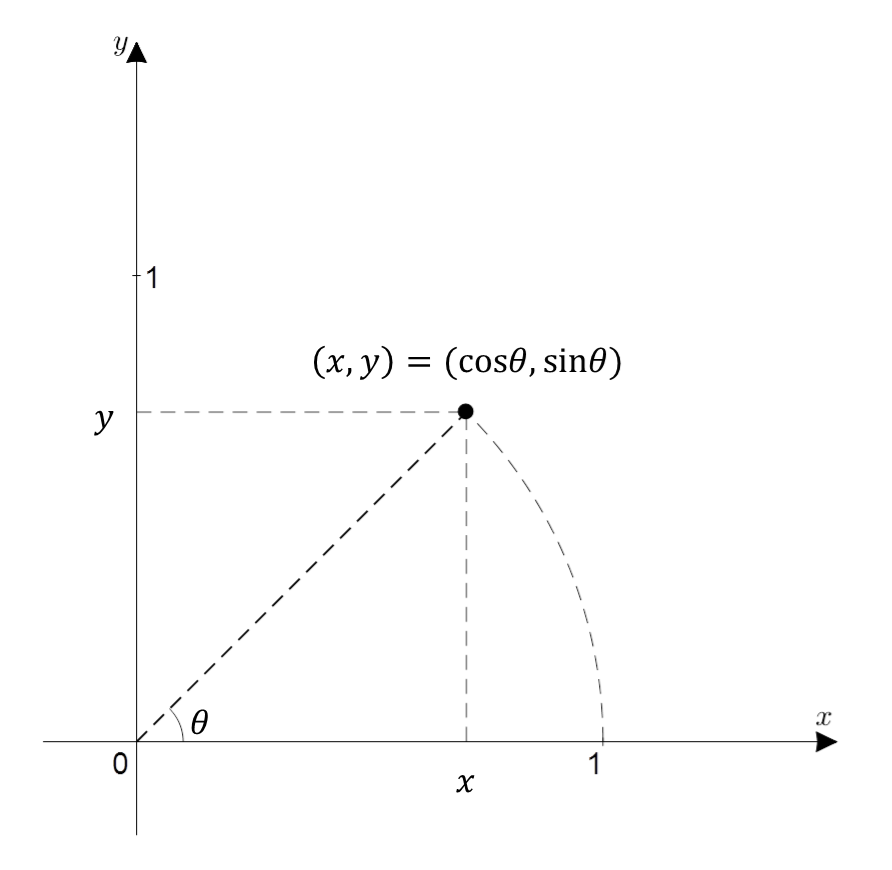Figure 1. The expression x + iy represented on the complex plane. When expressed in terms of trigonometric functions, it is cos(theta) + i sin(theta), where theta is the angle from the x-axis in radians.

## From the left-hand side of the formula

Now, let’s consider the left-hand side of equation (1). Ultimately, we have $e^{i\theta}$, which is a complex number, and this complex number represents a value that lies on the complex plane, rotating $\theta$ radians counterclockwise from the real axis.

First, let’s think about the definition of the natural constant $e$. The natural constant $e$ is defined as follows:

$e = \lim_{n\rightarrow \infty}\left(1+\frac{1}{n}\right)^n$

Then, how did we think about the case where the exponent $x$ is attached to the natural constant $e$…?

$e^x = \lim_{n\rightarrow \infty}\left(1+\frac{x}{n}\right)^n = \left[\lim_{n\rightarrow \infty} \left(1+\frac{x}{n}\right)^{n/x}\right]^x$

Now, if we carefully think about equation (4), we can consider that attaching the imaginary exponent $i$ to the natural constant $e$ can be thought of as follows:

$e^i = \lim_{n\rightarrow \infty}\left(1+\frac{i}{n}\right)^n = \left[\lim_{n\rightarrow \infty} \left(1+\frac{i}{n}\right)^{n/i}\right]^i$

If we separate the middle part of equation (5) and look at it separately, it becomes the following equation:

$\lim_{n\rightarrow \infty}\left(1+\frac{i}{n}\right)^n$

Let’s carefully think about the meaning of this equation (6) step by step.

### Imaginary Exponent Attached to the Natural Constant: Point on an arc

In equation (6), we made $n$ infinitely large, but let’s first consider the case where $n$ is 1.

First, when $n = 1$, the following holds:

$\text{n=1 }\Rightarrow \left(1+\frac{i}{1}\right)^1$

In other words, this represents the complex number $1+i$. If we represent it graphically, it would look like the following: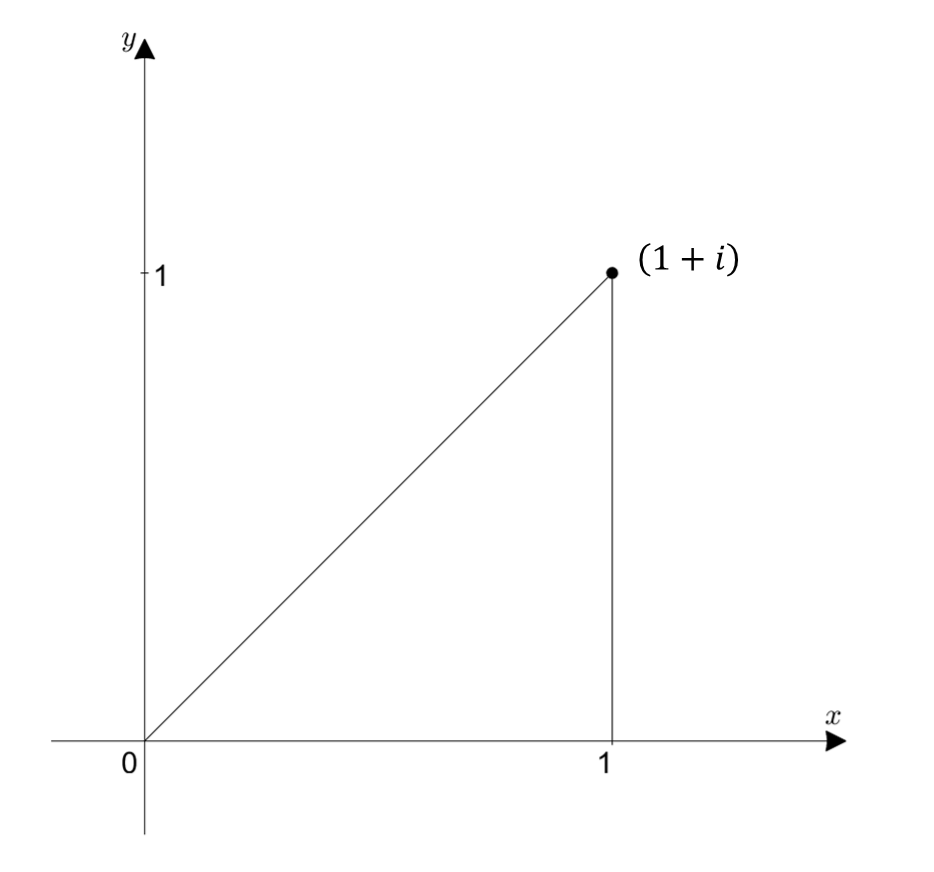Figure 2. The position of $1+i$ on the complex plane.

Let’s consider the case where $n = 2$.

$\text{n=2 }\Rightarrow \left(1+\frac{i}{2}\right)^2 = \left(1+\frac{i}{2}\right)\left(1+\frac{i}{2}\right) = 0.75+i$

How can we easily understand $\left(1+\frac{i}{2}\right)\left(1+\frac{i}{2}\right)$?

In simple terms, we can understand $\left(1+\frac{i}{2}\right)\left(1+\frac{i}{2}\right)$ geometrically by drawing a right triangle obtained by plotting $(1+i/2)$ and another right triangle with the hypotenuse of half the length of the hypotenuse of the first triangle as the height.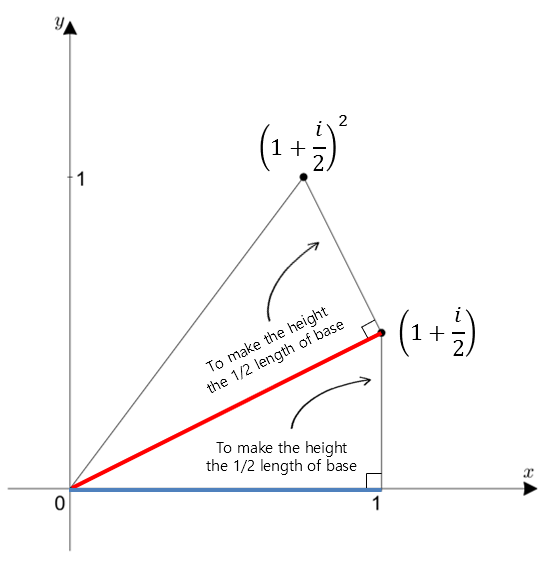Figure 3. Geometrical representation of the position of $(1+i/2)^2$ in the complex plane

Similarly, we can understand the case when $n = 3$ as follows: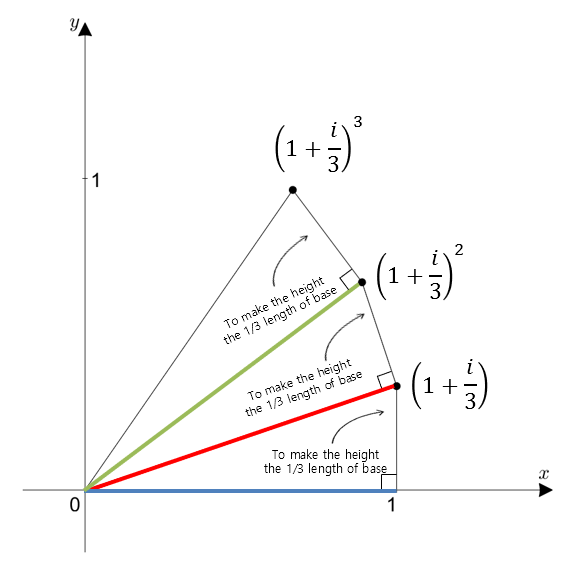Figure 4. Geometrical representation of the position of $(1+i/3)^3$ in the complex plane

Using the same method, we can consider the case when $n = 10$: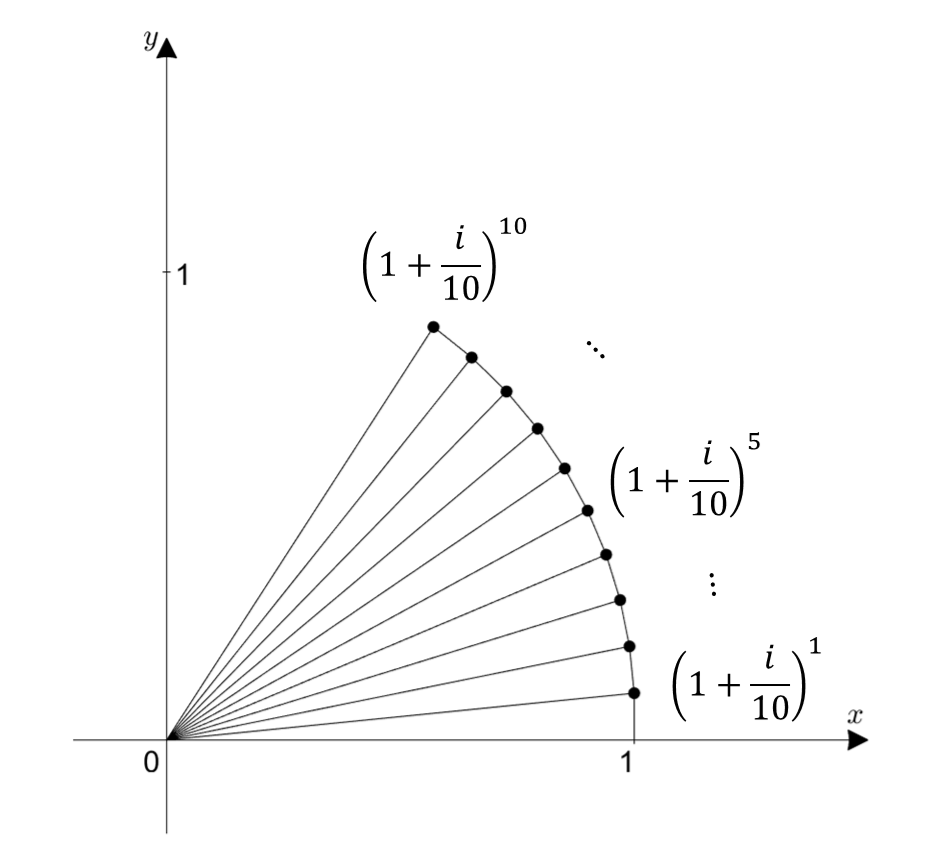Figure 5. Geometrical representation of the position of $(1+i/10)^10$ in the complex plane

As $n$ becomes larger, the hypotenuse of the right triangle with base and height of 1 and $1/n$ respectively becomes close to 1.

As $n$ approaches infinity, the point on the complex plane representing the value of Equation (6) becomes closer to the point on the arc with radius 1 and length 1.

Figure 6. As $n$ approaches infinity, the value of Equation (6) represents a point on the arc with radius 1 and length 1.

So, what does Equation (6) ultimately mean?

When considering the meaning of radians (radian) as described in this link, it refers to a point on an arc with a radius of 1 and an angle of 1 radian.

So how can we understand $e^{i\theta}$?

It represents a point on a circle with a radius of 1 and an angle of $\theta$ radians, and it can be expressed in another form as $\cos(\theta) + i\sin(\theta)$.

In this way, we can understand the geometric meaning of equation (1).

### The Meaning of the Natural Constant -> Growth through Rotation

Then, how can we interpret the inherent meaning of the natural constant e, which signifies “growth” as described in this link, in this context?

In my opinion, the natural constant has traditionally represented continuous growth in the same direction. However, with the introduction of imaginary exponentials, it can be understood as representing growth in a perpendicular direction.